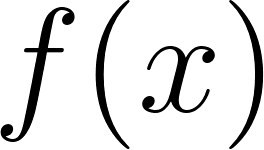×
Get Full Access to Calculus: Early Transcendentals - 1 Edition - Chapter 1.4 - Problem 9e
Get Full Access to Calculus: Early Transcendentals - 1 Edition - Chapter 1.4 - Problem 9e

×

Explain why the domain of the sine function must beISBN: 9780321570567 2

Solution for problem 9E Chapter 1.4

Calculus: Early Transcendentals | 1st Edition

• Textbook Solutions
• 2901 Step-by-step solutions solved by professors and subject experts
• Get 24/7 help from StudySoup virtual teaching assistantsCalculus: Early Transcendentals | 1st Edition

4 5 1 238 Reviews
19
2
Problem 9E

Explain why the domain of the sine function must be restricted in order to define its inverse function.

Step-by-Step Solution:

Step 1 of 3must be one to one on its domain for an inverse function to exist.

Step 2 of 3

Step 3 of 3

ISBN: 9780321570567

This full solution covers the following key subjects: function, its, explain, define, Inverse. This expansive textbook survival guide covers 85 chapters, and 5218 solutions. Since the solution to 9E from 1.4 chapter was answered, more than 402 students have viewed the full step-by-step answer. The full step-by-step solution to problem: 9E from chapter: 1.4 was answered by , our top Calculus solution expert on 03/03/17, 03:45PM. Calculus: Early Transcendentals was written by and is associated to the ISBN: 9780321570567. This textbook survival guide was created for the textbook: Calculus: Early Transcendentals, edition: 1. The answer to “?Explain why the domain of the sine function must be restricted in order to define its inverse function.” is broken down into a number of easy to follow steps, and 18 words.

Unlock Textbook Solution